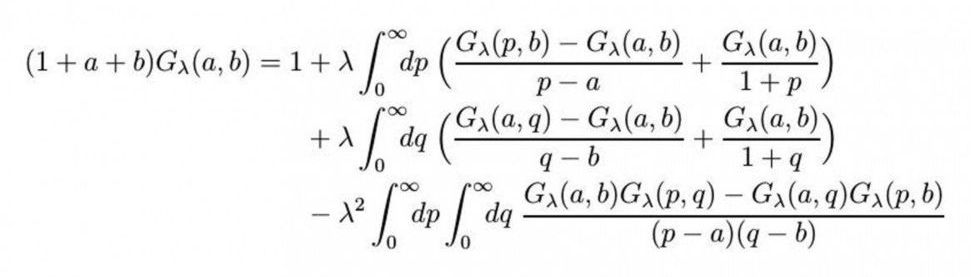## BlogCirca 2018

After 10 years, Prof. Raimar Wulkenhaar from the University of Münster’s Mathematical Institute and his colleague Dr. Erik Panzer from the University of Oxford have solved a mathematical equation which was considered to be unsolvable. The equation is to be used to find answers to questions posed by elementary particle physics. In this interview with Christina Heimken, Wulkenhaar looks back on the challenges encountered in looking for the formula for a solution and he explains why the work is not yet finished.

You worked on the solution to the equation for 10 years. What made this equation so difficult to solve?

It’s a non-linear integral equation with two variables. Such an equation is so complex that you do actually think there can’t possibly be any formula for a solution. Two variables alone are a challenge in themselves, and there are no established approaches for finding a solution for non-linear integral equations. Nevertheless, again and again during those 10 years there were glimmers of hope and as a result, and despite all the difficulties, I thought finding an explicit formula for a solution – expressed through known functions – was actually possible.